# CT81-5KV-Y5U-122M高压瓷片电容

## CT81-5KV-Y5U-122M高压瓷片电容详细参数

• ### CT81-5KV-Y5U-122M高压瓷片电容详细介绍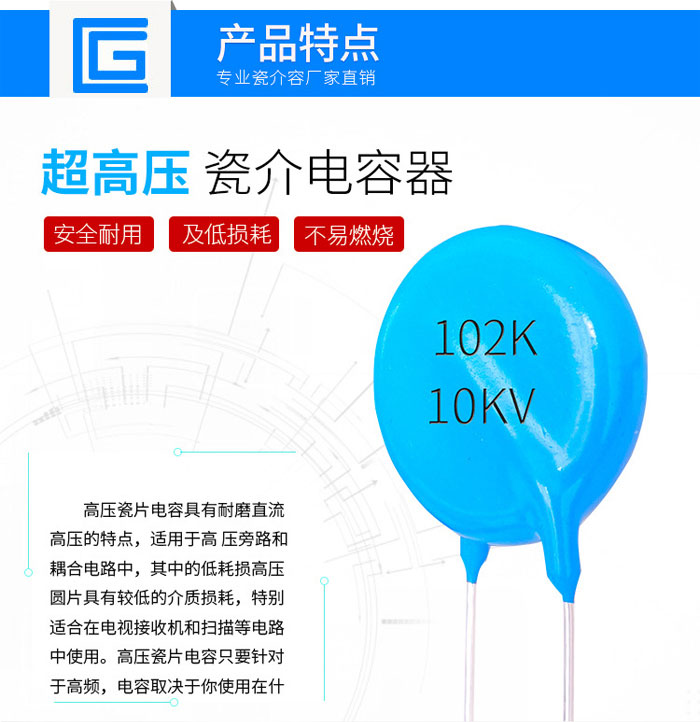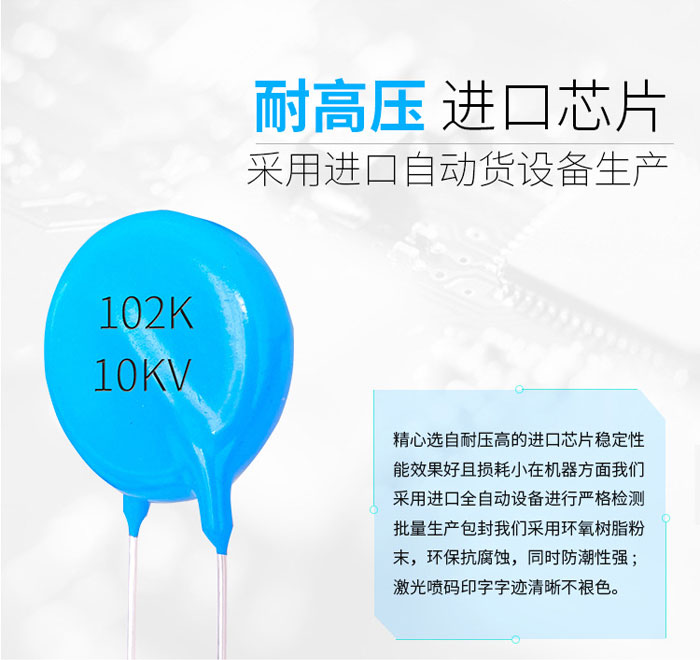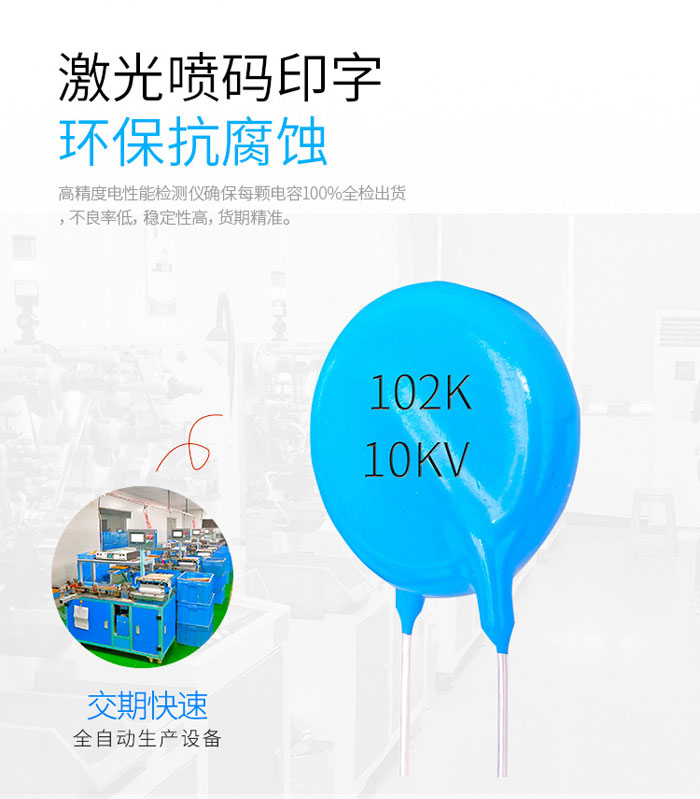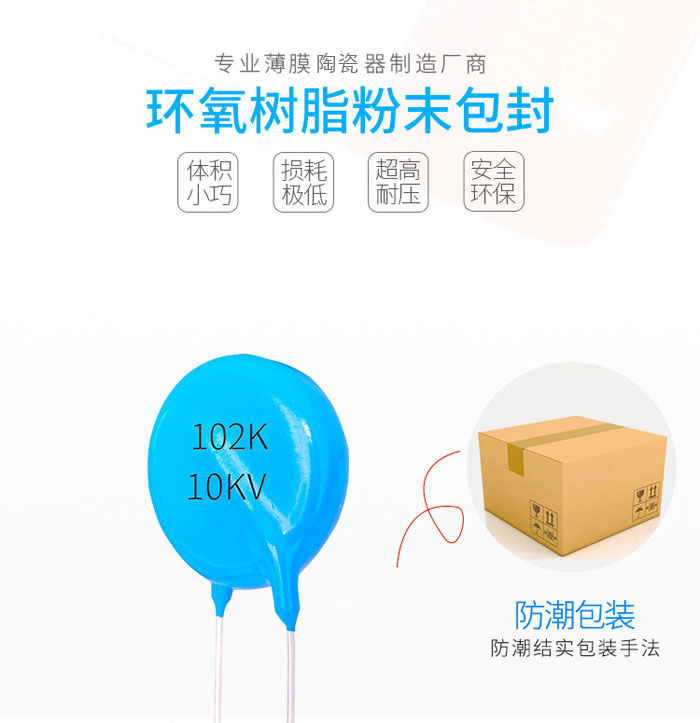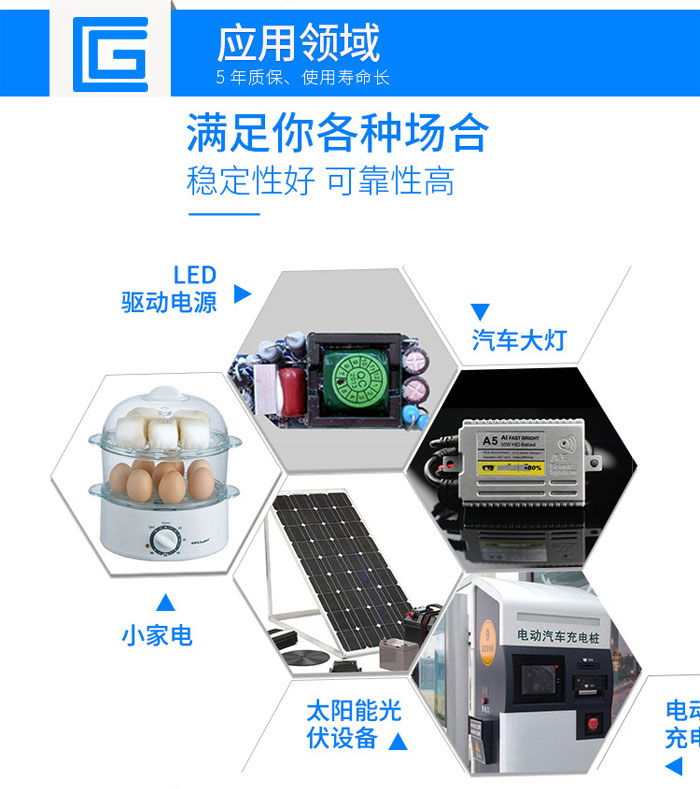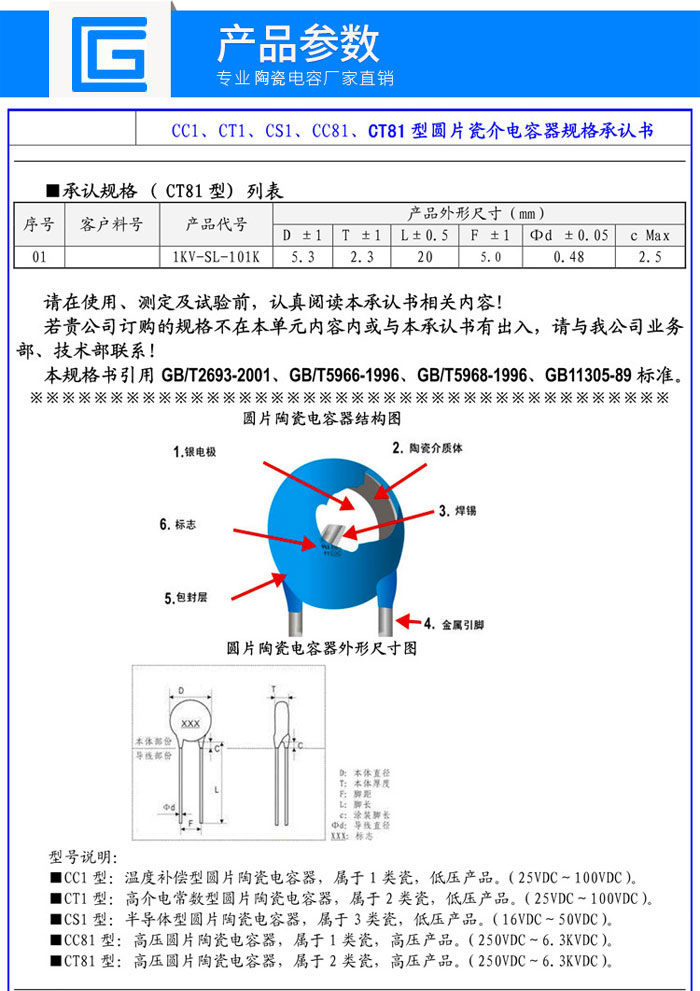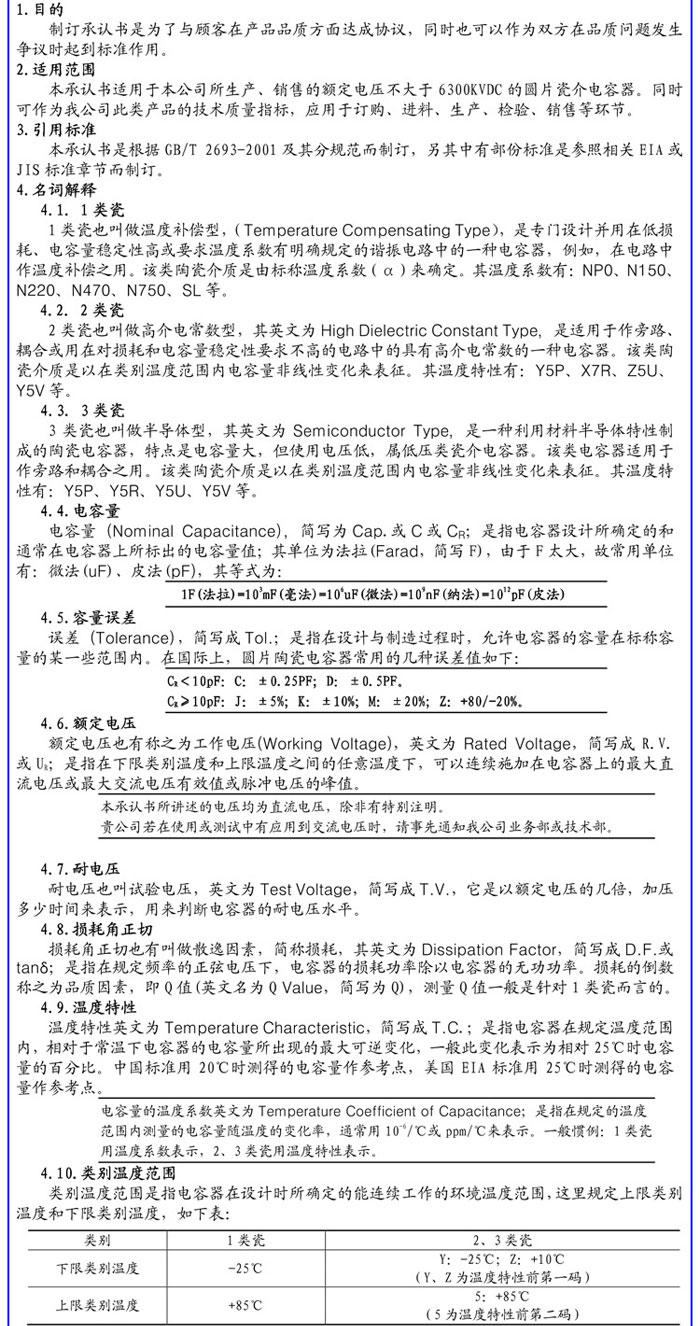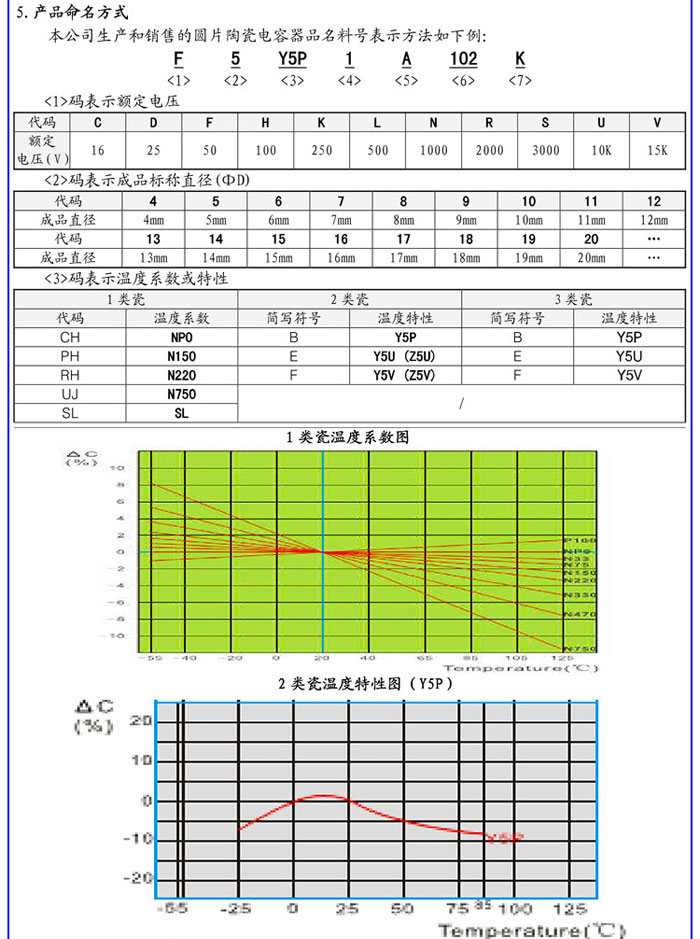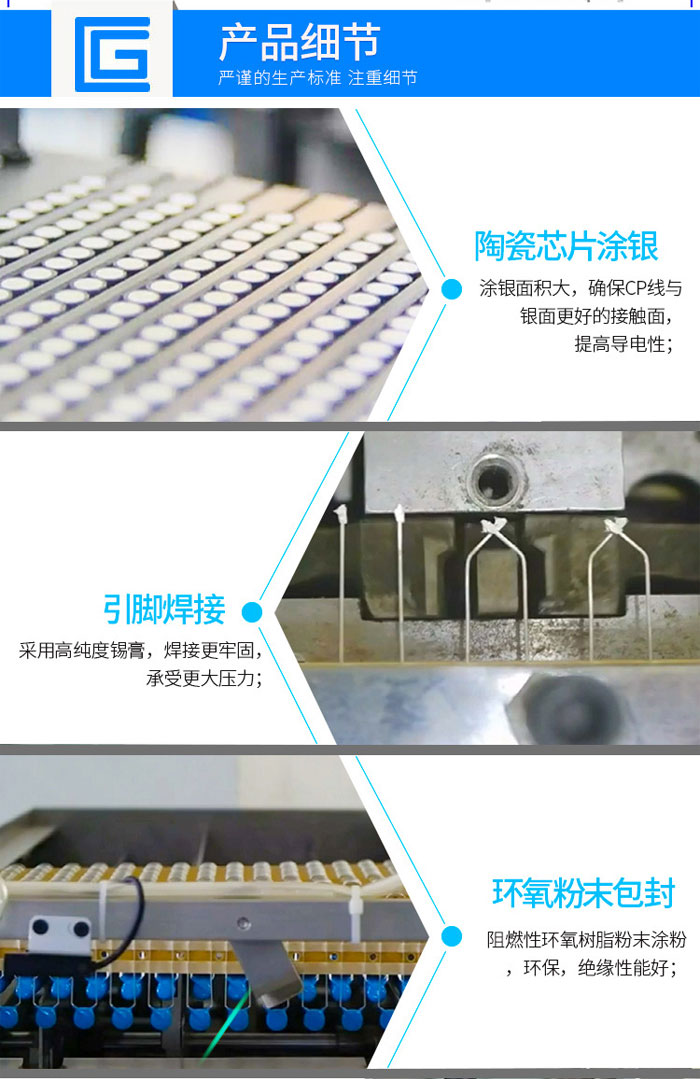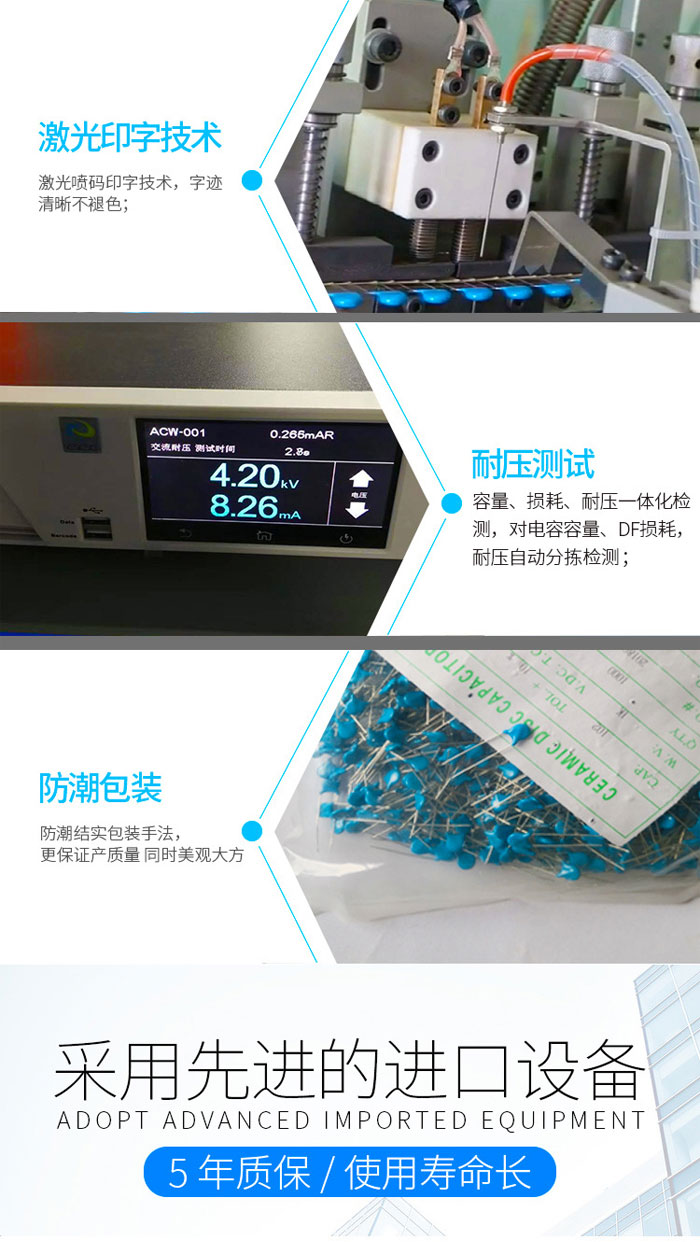• ### CT81-5KV-Y5U-122M高压瓷片电容相关信息

1、容量大的电容其容量值在电容上直接标明，如10 /16V；

2、容量小的电容其容量值在电容上用字母表示或数字表示；

1F=10^6uF=10^12

1F＝1000000µF

1m=1000

1P=1（如470P=470）

1P2=1.2

1n=1000；

102表示标称容量为10×(10^2)=1000= 0.001 µF；

103表示标称容量为10×(10^3)=10000= 0.01 µF；

104表示标称容量为10×(10^4)=100000= 0.1 µF；

105表示标称容量为10×(10^5)=1000000= 1 µF；

470表示标称容量为47；

223表示标称容量为(22×(10^3))（即22000）；

224表示标称容量为(22×(10^4))（即220000）=0.22uF

### 高压瓷片电容器Product categories

#### 相关资讯0769-85751806 全国服务热线

Q Q：120795732